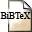# Algorithms in convex analysis to fit lp-distance matrices

## Abstract

We consider the MDS problem of fitting an l_p-distance matrix to a given dissimilarity matrix with respect to the weighted least squares loss function (STRESS). The problem is reduced to the maximization of a ratio of two norms on a finite dimensional Hilbert space. A necessary condition for a point where a local maximum is attained constitutes a nonlinear eigenproblem in terms of subgradients. Explicit expressions for the subgradients of both norms are derived, a new iterative procedure for solving the nonlinear eigenproblem is proposed, and its global convergence is proved for p in [1,2].

## BibTEX Reference Entry```@article{MaMe94,
author = {Rudolf Mathar and Renate Meyer},
title = "Algorithms in convex analysis to fit {\$l\sb p\$}-distance matrices",
pages = "102-120",
journal = "Journal of Multivariate Analysis",
volume = "51",
year = 1994,
hsb = RWTH-CONV-223133,
}
```Download bibtex-file